# How to split a long report into separate fields

Quite often we come across a “long report text” in a single field and have to split it into multiple lines, for example: To match the correct field length in an OBX-5 field. We created the helper function `splitText()` to split any type of ASCII text into shorter lines.

Tip: The `splitText()` function can be re-used any time you want to split up a string of ASCII text, into shorter lines of a specified length. As always Iguana is flexible, so if the function does not quite match your needs then you can adapt it.

In this example we take the original “long report text” from the original single OBX-5 field. Then we split it into chunks with a maximum length of 80 characters (the HL7 specified maximum length for an OBX-5 field). We make the split the at the last complete word not exceeding 80 characters. All this is done without changing the text, not even original “new lines”. Each chunk is then placed in the OBX-5 field of a an OBX segment. In this example the text is split into six lines so we will end up with six OBX repeats in our HL7 message.

New lines in reports can be indicated in several ways:

• As a “~”, which is (mis)interpreted as a repetition of an OBX-5 field
• As a <CR>
• As an <LF>
• As a <CR><LF> combination

We preserve the content for each of these cases.

This is our original data in text format: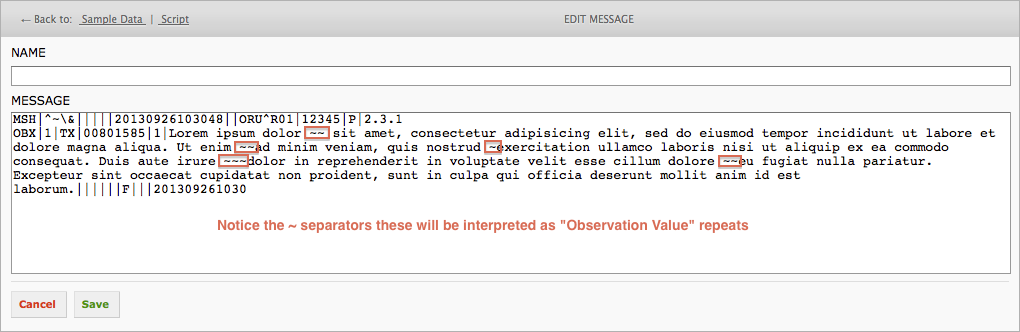This is our original data in an HL7 node tree: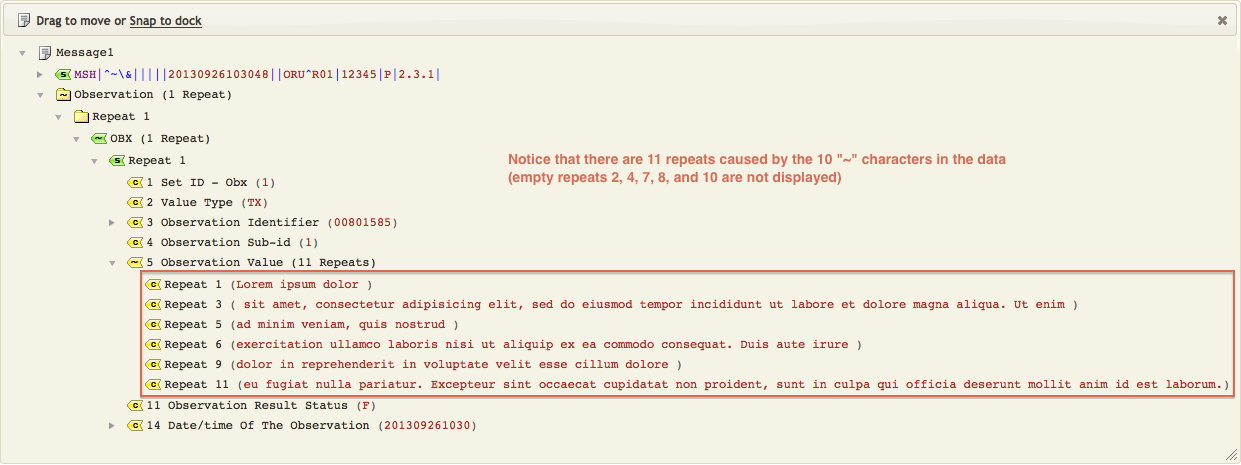This is the final result as a node tree:This is the result as text: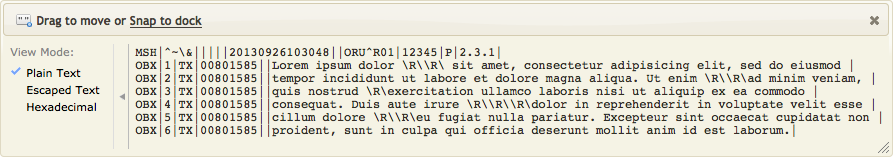This project contains the code and sample data split_text_Translator.zip

This is the `splitText()` function: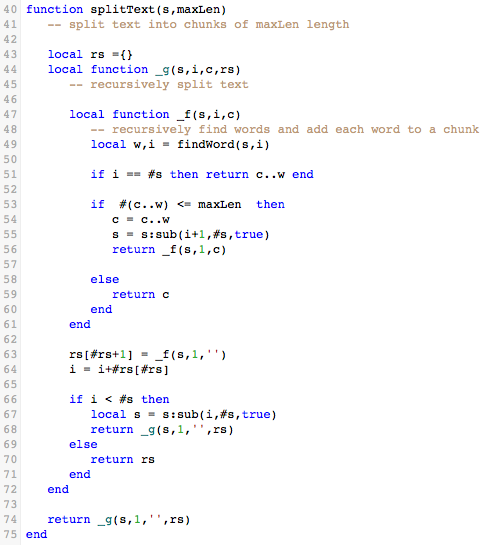This is the ` findWord()` helper function, called from `splitText()`:This is the complete example of how we call it from `main()` to add OBX segments to our sample HL7 message: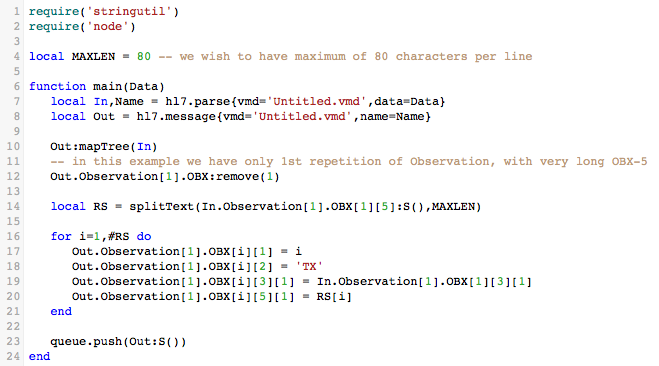One of our customers raised an issue in our forum that this code may fail  when splitting large messages. This is because the `splitText()` function above is recursive and it is calling itself more than 100 times. To resolve this issue you can use the non-recursive version of the code below.

This is the error:This is the code rewritten without recursion:

```require('stringutil')

local MAXLEN = 80 -- we wish to have maximum of 80 characters per line

function main(Data)
local In,Name = hl7.parse{vmd='Untitled.vmd',data=Data}
local Out = hl7.message{vmd='Untitled.vmd',name=Name}

Out:mapTree(In)
-- in this example we have only 1st repetition of Observation, with very long OBX-5
Out.Observation.OBX:remove(1)

local RS = splitText(In.Observation.OBX:S(),MAXLEN)

for i=1,#RS do
Out.Observation.OBX[i] = i
Out.Observation.OBX[i] = 'TX'
Out.Observation.OBX[i] = In.Observation.OBX
Out.Observation.OBX[i] = RS[i]
end

queue.push(Out:S())
end

function splitText(s,maxLen)
local rs ={}
local start = 1
local i = 1

while true do
local cnt = s:sub(start, start + maxLen):reverse():find('[ \t]')
if cnt == 1 or cnt == 2 then
rs[i] = s:sub(start, start + maxLen - 1)
start = start + maxLen
i = i + 1
else
if #s:sub(start) <= maxLen then -- catch last rep
rs[i] = s:sub(start)
break -- exit loop on last rep
else
cnt = cnt - 1 -- adjust cnt to = find in string of maxLen length
rs[i] = s:sub(start, start + maxLen - cnt)
start = start + maxLen - cnt + 1
end
i = i + 1
end
end
return rs
end

```